In the process of creation of Network diagram, several books and reference material talks about various ways to calculate Early Start (ES) for activity node, like some suggest to start ES of the first activity with 0 and others suggest it to start with 1. This tends to create confusion among the students preparing for PMP® certification.

For instance, in PMBOK® Guide Sixth Edition (p. 177, Fig 6-18) the network diagram is drawn with 1 as ES for the first activity on the path and there are other reference books which have used 0 as ES for the first activity.

In this blog, I intend to explain the methods, their similarities and differences. The method in which ES of first activity is taken as 0, referred as ‘Zero Method’ and where the ES of first activity is taken as 1, referred to as ‘One Method’.

Let’s begin with the similarities between these 2 approaches first

1. When we calculate Critical Path using either of these approaches, it will be same. Critical Path stays uninfluenced, as it is a sum of durations of all activities on the path.
2. No difference in calculation of Float of the path or activities, as it is the difference between LF and EF (LF—EF) or LS and ES (LS-ES), irrespective of the way ES and EF are calculated.
3. Either we use Zero method or One method to calculate early start, Finish values of the activities will be same.

Now, let’s begin with the differences between these two methods

1. It is the start values that differ depending on the method selected
2. The way calculation is done for start values

Let us calculate the start and finish of all activities given in below table using both methods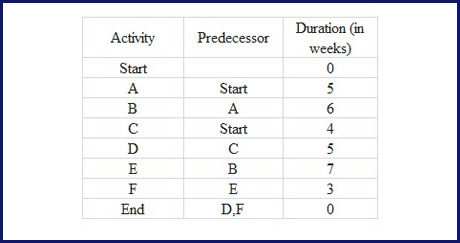The PDM of the above activities: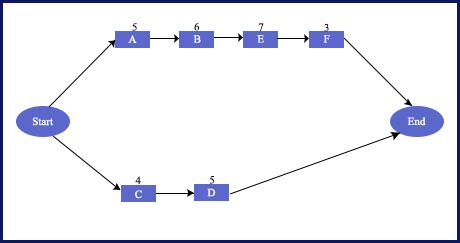We can see here that there are two paths in the above diagram.

1. Start-A-B-E-F-End, and duration of this path (sum of all activities) = 5 + 6+ 7 + 3 = 21
2. Start – C-D-End, duration of this path = 4 + 5 = 9

The Critical Path = Path of the Longest duration

And, if we compare duration of both paths, Start-A-B-E-F-End has got larger total duration than the other path.

We can see here that calculation of Critical Path is not dependent on the way we choose to calculate Start or Finish of the activities.

Let us Calculate ES and EF of activity node a using zero method.

ES = 0 (as A is the first Activity on path)

EF = ES + Duration = 0 + 5 = 5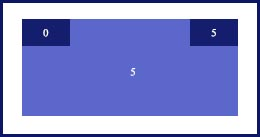If we use one method to calculate ES and EF of Activity A

ES = 1

EF = ES + Duration – 1 i.e.  EF of A = 1 + 5 -1 = 5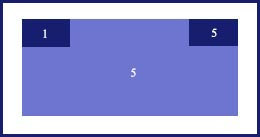In case of One method, is it assumed that when we calculate start of the first activity of path,activity starts right from the beginning of the 1st day or ES day and complete on the end of EF day.

So here, we can see that Finish value (EF) is same in both the methods. ES of successor activity

In case of Zero method, when we calculate ES of successor activity, it is same as the value of EF of predecessor activity.

So, if we calculate ES of Activity B, using zero method, its

ES of Activity B = EF of predecessor activity = 5

EF of Activity B = ES + Duration = 5 + 6 = 11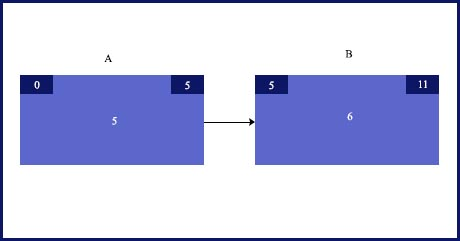In case of one method, when we calculate ES and EF of Activity B

ES of Activity B = EF of predecessor activity + 1 = 5 + 1 = 6

EF of next activity = EF + Duration -1 = 6 + 6 -1 = 11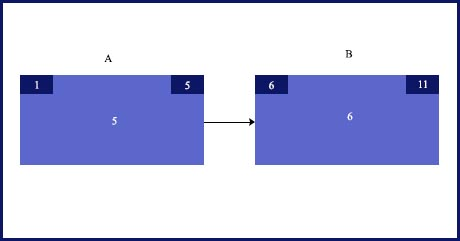So here, we have seen that the ES value of predecessor nodes is different in both the methods.

Let us calculate ES, EF, LS and LF of whole project using Zero method,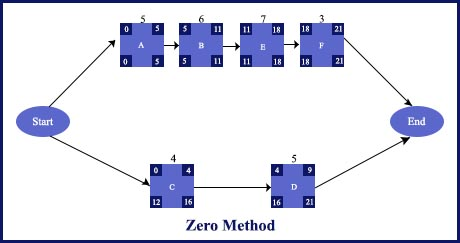And, now ES, EF, LS and LF of whole project using one method: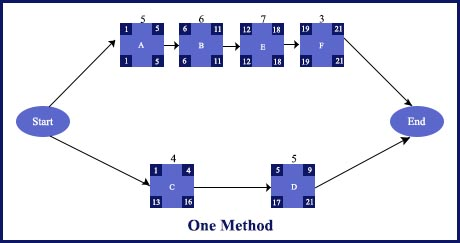Note: There is NO right or wrong way of starting ES with 0 or 1, these are just different approaches. Even PMBOK® Guide Sixth Edition ((Page# 177) states that there are other accepted conventions (from using 1 as the calendar start of the first activity of path) which can be used for calculating calendar start of the activities.

Now, though many students found zero methods easier to follow. For calculation of critical path or float or finish values you can use either method. But, for calculation of Start values, practice both the methods.

You can join the discussion on the same in our here Discussion Forum. You can also log into our YouTube channel watch the videos on the related topics.

```Enroll to our FREE PMP® Introductory Program to learn more about PMP® certification
```